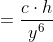# Introduction to Exponents - Rules of Exponents

Source: Online Math Learning

Since we often see exponents throughout all math courses, it is important to understand the rules of exponents. We need to understand how to distribute, add, multiply and divide exponents in order to simplify expressions or manipulate equations that have exponents. The rules of exponents, like those involving multiplication of terms, are important to learn and will be used throughout Algebra I and II and Calculus. How to understand the vocabulary of exponents. Understanding and working with exponents by examining academic vocabulary of various problems involving exponents.

## Exponents Rules

The following table gives the Rules of Exponents.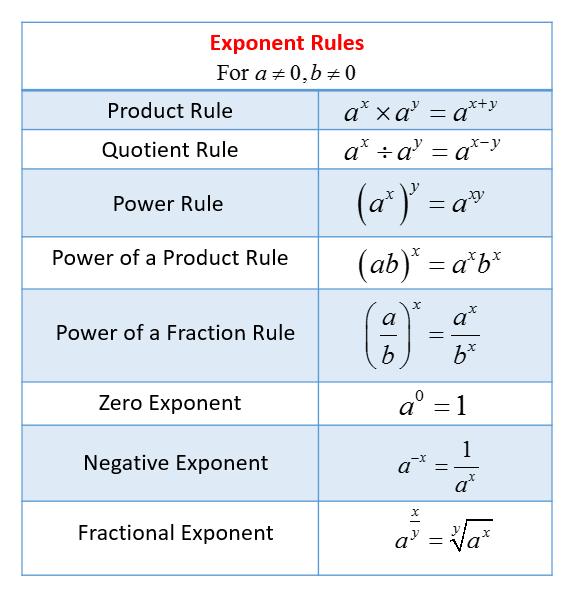There are different rules to follow when multiplying exponents and when dividing exponents. If we are multiplying similar bases, we simply add the exponents. If we are dividing, we simply subtract the exponents. If an exponent is outside the parentheses, it is distributed to the inside terms. It's important to understand the rules of multiplying exponents so that we can simplify expressions with exponents.

## Examples

Some complex expressions can be simplified by using the above rules. Take the following example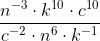Swap the negative exponents from numerator to denominator and vice-versa.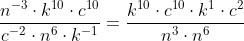Add the exponents of the products with the same base: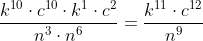Take the next example: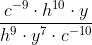Swap the terms to make the exponents positive: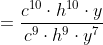Subtract the exponents of the similar bases: